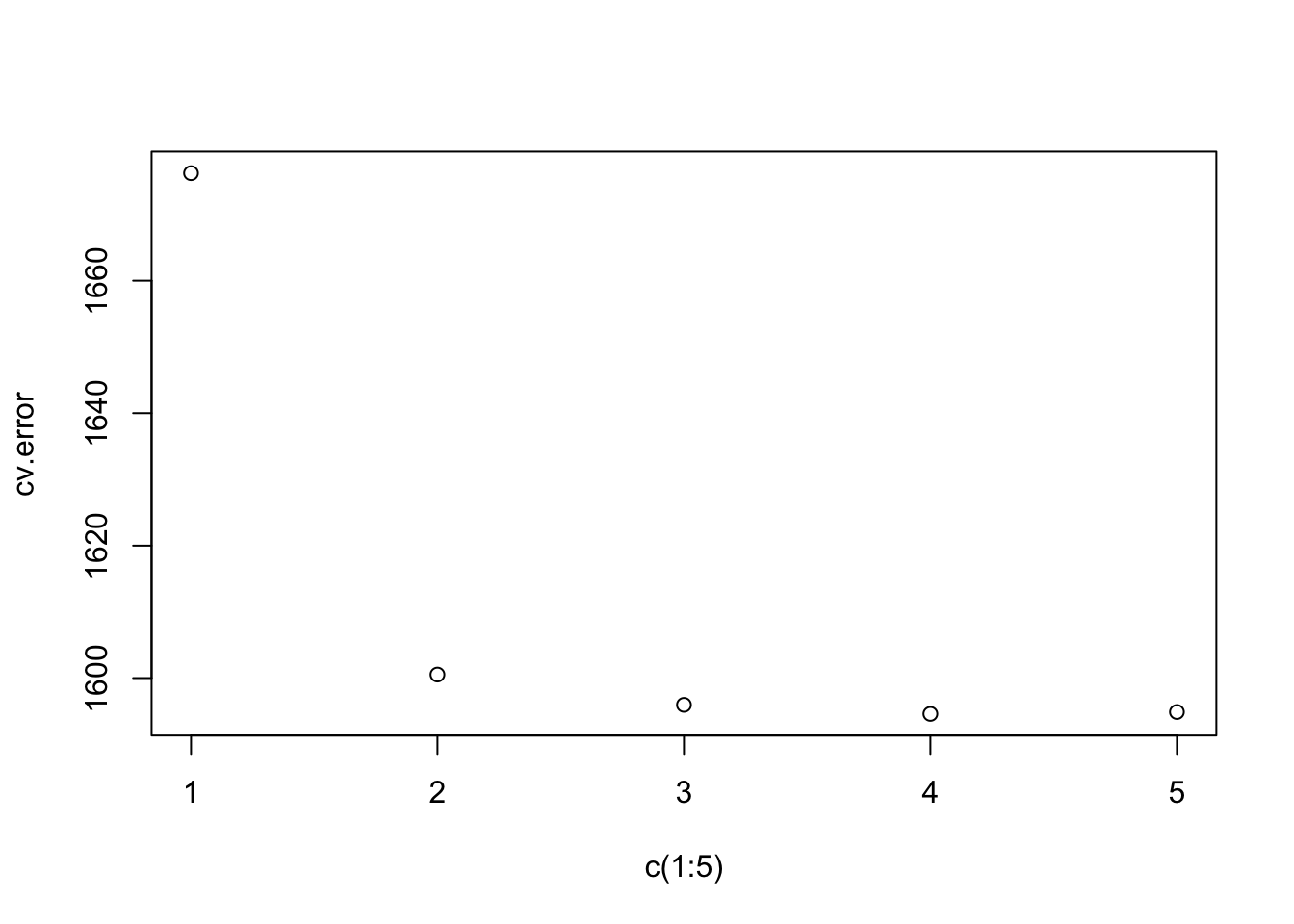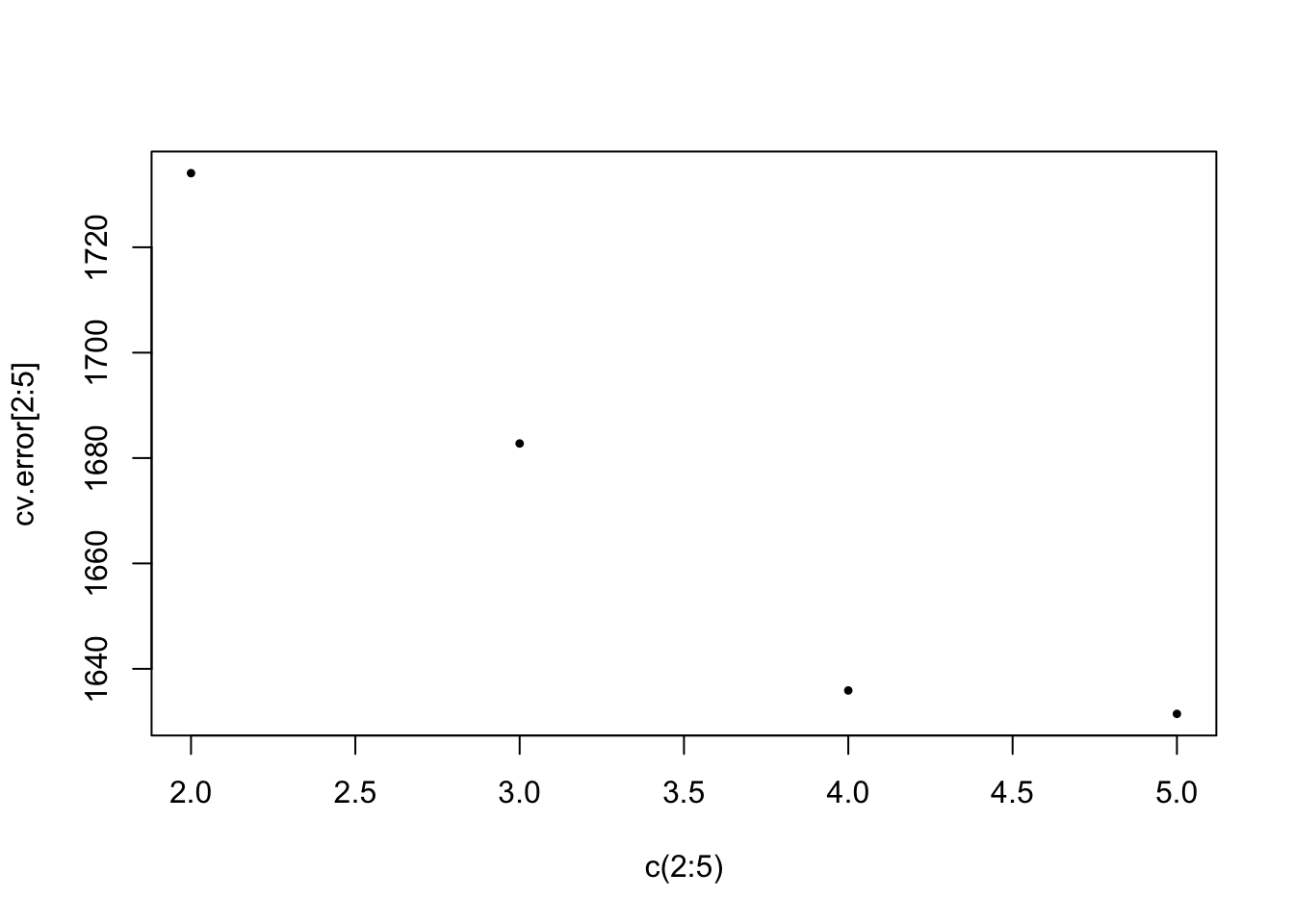ISLR Home

# Question

p299

In this exercise, you will further analyze the Wage data set considered throughout this chapter.

1. Perform polynomial regression to predict wage using age. Use cross-validation to select the optimal degree d for the polyno- mial. What degree was chosen, and how does this compare to the results of hypothesis testing using ANOVA? Make a plot of the resulting polynomial fit to the data.

2. Fit a step function to predict wage using age, and perform cross-validation to choose the optimal number of cuts. Make a plot of the fit obtained.

library(ISLR)
library(boot)

# 6a

# Creating a placeholder for the cv errors
cv.error = rep(0, 5)

# Running a for loop to iterate through each polynomial and fitting data
for (i in 1:5) {
# Fitting data the polynomial i
glm.fit = glm(wage ~ poly(age, i), data=Wage)

# Saving the CV estimate for the fit
cv.error[i] = cv.glm(Wage, glm.fit)$delta } ## Plot CV Error # Plotting the results of for loop plot(c(1:5), cv.error)Comments: • Based on the one-SE rule, the cv chooses the 3rd degree polynomial • The ANOVA chooses 4th degree polynomial # 6b cv.error = rep(0, 5) # Running a for loop to iterate through each step function and fitting data for (i in 2:5) { # Fitting data the polynomial i print(i) Wage$age.cut = cut(Wage$age, i) glm.fit = glm(wage ~ age.cut, data=Wage) # Saving the CV estimate for the fit cv.error[i] = cv.glm(Wage, glm.fit)$delta
}
##  2
##  3
##  4
##  5

## Plot CV Error

plot(c(2:5), cv.error[2:5], pch=20, cex=0.5, lwd=2)